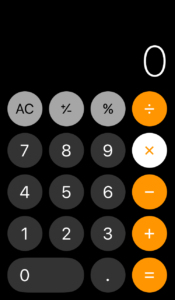# Design Critique: Calculator (iPhone Application, iOS 11.2.5 version)The calculator application simply defined is a digital calculator. It can perform the most basic mathematical functions (such as addition/subtraction) all the way to solving more complex algebraic problems (such as logarithmic functions). For brevity, I will mainly focus upon the interface attributes that are present in the basic calculator mode (Refer to Figure 1 for images of basic and advanced calculator interfaces).

Figure 1. Basic (left) and Advanced (right) calculator interfaces for iPhone iOS 11

In terms of overall discoverability, the calculator app is excellent. The use of Gestalt Principles (“button” shape, coloring, and foreground placement of the numbers and mathematical operators) provides excellent examples of effective signifiers that positively influence the discoverability of the possible functions of the app. Another example of good discoverability is the fact that all but one of the possible functions is present on the screen at all times (refer to Figure 2 for this feature).

Figure 2. C/AC Functions

The C/AC functions both “clear” the calculator with C only clearing the last action performed and AC clearing all actions. The labeling of these functions with just letters may be confusing to people of different backgrounds (i.e. numbers are the same in almost every country but “C” and “AC” may represent other things depending on where you are). The labels and functions rely on knowledge of language, culture, and previous use of a similar calculator (knowledge in the head). It might be more beneficial to change the labeling to “clear” and “clear all”, although knowing what the difference between the two will still require knowledge in the head. However, this knowledge is made immediately apparent through feedback once a user has executed each function.

One major problem with the discoverability of the overarching function of the app (to carry out mathematical calculations) is that it relies almost entirely on the user’s prior knowledge, or as Norman calls it, knowledge in the head. If a user has never seen a calculator (physical or digital) before, they might have a harder time figuring out what this app is meant to do. The chance that a person who has an iPhone, doesn’t know what a calculator is, however is unrealistic. The slim chance that they have no prior knowledge of a calculator, the speed and accuracy of the feedback should quickly alleviate the user’s frustration/confusion, given that the user knows math and what numbers are. One example of feedback used in this app is the “highlight” or color change a mathematical operator presents once it is selected (Figure 3). This gives the user instant feedback that the operation they selected is active and puts the knowledge in the world of what function will be performed. The feedback provided on this app is sufficient for the user to easily navigate the Gulf of Evaluation.Figure 3. Active multiplication operator

One of the constraints in this application is the inability a user has to select two mathematical operators at once for the calculator to perform. This is a mathematical standard (i.e. “1 – ÷ 4” is not a possible equation) and providing this constraint prevents users from slips, and getting an error message instead of an answer.

The mapping of the operators and numbers does well to demonstrate the effectiveness of the Gestalt principle of grouping by similarity. The most prominent example of the use of similarity are the color differences of the functions. All of the numbers are a dark gray, the mathematical symbols are orange, and the “other” functions are a light gray. The use of color to suggest function similarity is an excellent example of putting knowledge in the world. Maybe someone doesn’t know “÷” represents the division function, but they do recognize “+” as the addition function. Based on the coloring and relative proximity of the buttons they could probably guess that “÷” is a mathematical operation in the same way that “+” is a mathematical operation. The effective mapping and use of constraints allows the user to effectively move through the Gulf of Execution.